## Making excellent histograms on your calculator

Your graphing calculator will make superb histograms from data you enter. If you have tried to do this, but your histograms don’t look the way you expect, follow this procedure. You have to tweak the x-min, x-max, and x-scale values to match your preferences. Let’s say that you have collected the following data (perhaps they are the heights of 30 people in your class):

61.0    61.5     62.0    65.0    65.5     65.5     66.0     66.0
66.5    66.5     67.0    67.0    67.5     68.0     68.5     68.5
69.0    69.0    69.0    69.5    69.5     70.0     70.5     72.0
72.0    72.0    74.0    74.0    75.0     75.5

 1. Determine the smallest class value and the class width. For this data set, I would display the data in five classes. We can set the smallest class value to 61, the largest class value to 76 and therefore the class width will be 3. 2. On your calculator, enter the data into a list. Here I’ve put it into L1.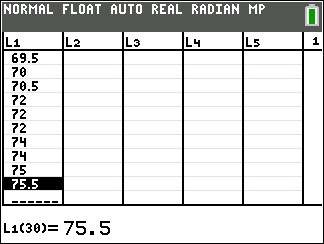3. Go to STAT PLOT and turn one of the plots on. 4. Select the histogram type and if necessary specify the Xlist location.5. Press zoom and select 9 (ZoomStat). Your graph probably won’t look right, but we’ll fix that. 6. Press the WINDOW button and set Xmin= to the smallest class value, Xmax= to the largest class value, and Xscl= to the class width. You might also need to adjust the Ymin and Ymax values.7. Press GRAPH, and admire your excellent histogram.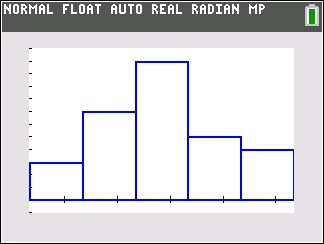## Using tree diagrams to find conditional probabilities

Those problems that ask you to find the probability of a series of events “without replacement” can be scary because the probabilities of each event keep changing. (These are known as conditional probability problems.) If the number of possible outcomes isn’t too large, you can tame these problems by using a tree diagram to simplify your calculations.

1. For the first event, draw a tree branch for each possible outcome.
2. At the end of each branch, draw a tree branch for each possible outcome of the second event.
3. Continue until you have a column for every event.
4. For every branch on the tree, write down the probability of that event occurring at that location.
5. Then multiply all the branches from first event to last event to find the probability of any one outcome.
6. Add various events together to get the probability of any compound outcome.

Here’s a simple example that shows how this process works. Let’s say you have a candy dish with 10 red candies, 15 green candies and 20 blue candies. You want to know the probability that you draw at least two red candies or at least two blue candies. There are a lot of different possibilities here, but a tree diagram simplifies everything greatly. Start by drawing a tree with every possible outcome (R, G and B in this example). Then from each outcome, draw another tree representing each outcome for the second draw. Repeat for the third draw. Your tree will look like this: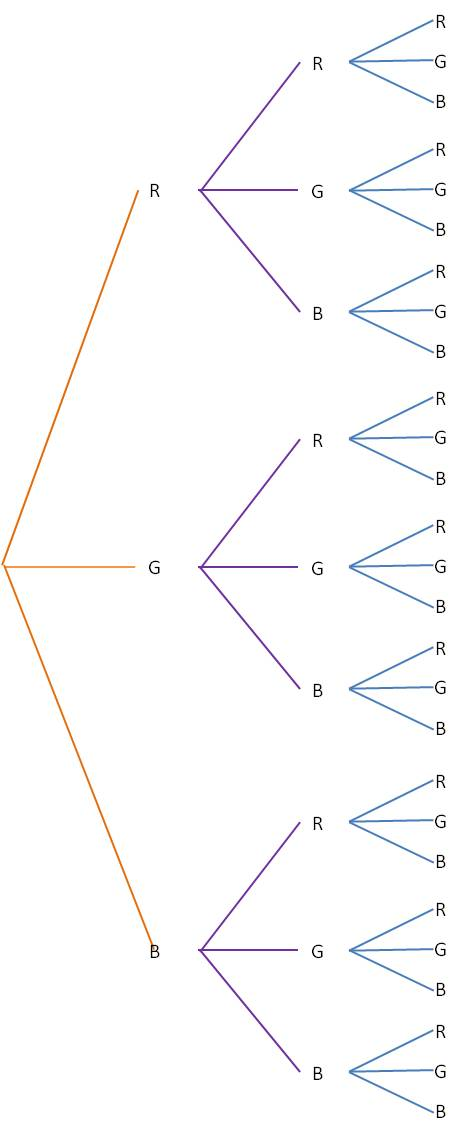(You can see that this process will get pretty unwieldy if there are too many outcomes or too many events.)

Next, label each branch with the probability for that outcome. Note that the probabilities change depending on which outcomes have already occurred. For our example, the tree would now look like this: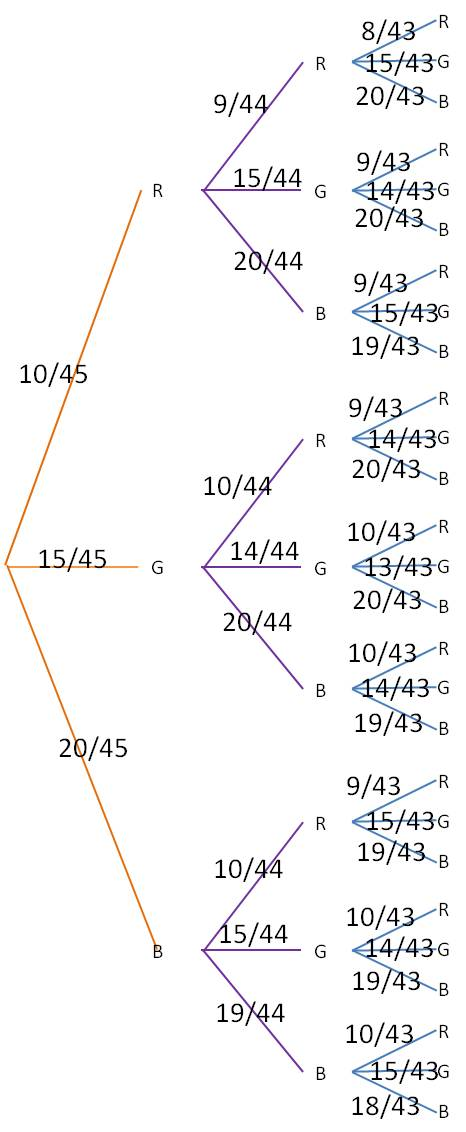Finally, for each of the branch ends at the right, multiply together all the probabilities leading to that endpoint. For example, the very top branch, which represents RRR, you would multiply 10/45*9/44*8/43 to find the probability of getting a red candy on all three draws. The final table looks like this (to make the table easier to read, we have calculated only those branches that represent at least two reds or at least two blues):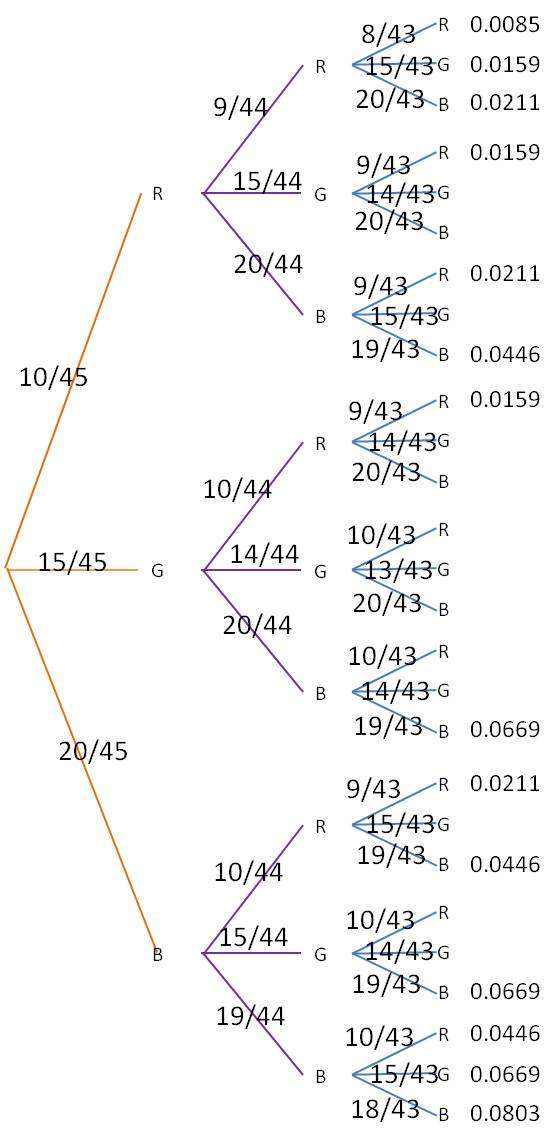The probability of our desired event is then the sum of all of listed probabilities: 0.5352.

## Calculating the probability of an event by its complement

You will often be asked to calculate the probability of a compound event; that is, an event that contains two or more simple outcomes. For example, if you flip five coins, what is the probability that you get either four or five heads? To solve this problem, you calculate the probability of getting exactly four heads and the probability of getting exactly five heads, then add the numbers together:$P(x=4 \text{ or } x=5)=P(x=4)+P(x=5)=$$\displaystyle \binom{5}{4} \! \left ( \dfrac{1}{2} \right )^4 \!\! \left ( \dfrac{1}{2} \right)^1+ \binom{5}{5} \! \left (\dfrac{1}{2} \right )^5 \!\! \left ( \dfrac{1}{2} \right )^0= \dfrac{5}{32}+ \dfrac{1}{32}= \dfrac{6}{32}= \dfrac{3}{16}$

But what if a compound event contains a lot of simple events? For example, if you roll ten dice, what is the probability you get at least two sixes? To solve this the way we did the previous example, we would need to find the probability of getting exactly two sixes, the probability of getting exactly three sixes, and so on, up to the probability of getting exactly 10 sixes. Although the calculations are not difficult, it is very tedious to find nine different probabilities in order to add them all together. For this problem, it is much simpler to calculate the complement of the given event. The complement of “at least two sixes” is “at most one six”. So calculate the probability of getting at most one six when you roll ten dice:$P(x=0 \text{ or } x=1)=P(x=0)+P(x=1)=$$\displaystyle \binom{10}{0} \! \left ( \frac{1}{6} \right )^0 \!\! \left ( \frac{5}{6} \right )^{10}+ \binom{10}{1} \! \left ( \frac{1}{6} \right )^1 \!\! \left ( \frac{5}{6} \right )^9=0.1615+0.3230=0.4845$

Because the probability of an event A and its complement (not A) add up to 1, the probability of getting at least two sixes is then$1-0.4845=0.5155.$

Calculating a compound probability by finding the probability of the complement instead is the basis of many problems in statistics. It’s up to you to determine when this is the best approach to solving a problem.

## The addition rule and multiplication rule in probability

Two of the many formulas you learn in statistics are referred to as the Multiplication Rule and the Addition Rule. How do you figure out when to use each one? This is actually a pretty easy one. The multiplication rule is used to determine the probability of two events both happening, one after the other. The key word to look for in a problem is ‘and’.

Example 1: You draw a single card from a standard deck, replace it and draw a second card. What is the probability you draw a seven first AND a heart second? Because you want the probability of both events occurring, you use the multiplication rule.

The addition rule is used to find the probability that either one of two events occurs. The key word here is “or’.

Example 2: You draw a single card from a standard deck, replace it and draw a second card. What is the probability you draw a seven on the first draw OR a heart on the second draw? Because you want the probability of either event occurring, you use the addition rule.

How do the rules work? The multiplication rule comes in two forms. If the two events are independent, that is, if the probability of the second event does not change when the first event occurs, then the formula is simple:$P(A \cap B) = P(A) \cdot P(B)$

If the probability of the second event depends on the first event occurring, the formula is modified slightly to show this:$P(A \cap B) = P(A) \cdot P(B|A)$

You can see from either formula why this is called the multiplication rule. The addition rule is just slightly more complicated.$P(A \cup B)=P(A)+P(B)-P(A \cap B)$

You can see from this formula why it is called the addition rule. Let’s solve both problems.

Example 1: The two events are independent, so we use the first version of the rule:$P(A \cap B) = P(A) \cdot P(B)=\dfrac{4}{52} \cdot \dfrac{13}{52}= \dfrac{1}{52}$

Example 2: (Note that we use the result from Example 1 in solving this problem.)$P(A \cup B)=P(A)+P(B)-P(A \cap B)=\dfrac{4}{52}+ \dfrac{13}{52}- \dfrac{1}{52}= \dfrac{16}{52}= \dfrac{4}{13}$

## Calculating probabilities as a fraction

The first day you are asked to find probabilities, the questions are relatively simple:

• What is the probability of rolling a 2 or a 3 on a standard die? [Ans: 2/6 = 1/3]
• What is the probability of drawing a five from a standard deck of cards? [Ans: 4/52 = 1/13]

But very quickly, the problems get a lot more complicated:

• What is the probability of flipping a coin ten times and getting exactly 8 heads?
• What is the probability of being dealt a full house (three of a kind plus a pair) in a five card poker hand?
• A class has 14 boys and 12 girls. If the teacher randomly creates a new seating chart, what is the probability that the six students in the front row comprise exactly four boys and two girls?

How do you attack these problems? The best way is to think of probability as a fraction. The numerator counts all the ways the specified event can occur, and the denominator counts all the ways any event can occur:

Any event

Then you use combinatorics to count all the possibilities on the top and on the bottom. Let’s see how we can use this method to answer the three questions posed above:

(Note that this question could also be treated as a binomial probability and solved that way.)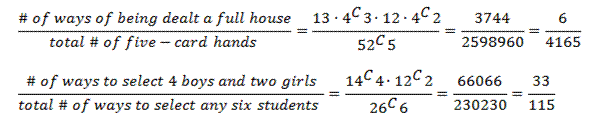## Identifying a probability distribution II—normal, t and chi-square

Often the first step in determining the probability of an event is determining the probability distribution to which the event belongs. Good statisticians can identify the correct distribution right away; if you learn some simple rules, you can specify the correct distribution just like the experts. In this post, I describe how to identify the three most common continuous distributions: normal, t and chi-square.

Requirements for a normal distribution

Many times you will be told when a distribution is normally distributed. When you are conducting hypotheses tests, you will often assume that the distribution is normal (or at least approximately normal) when the following conditions hold:

• The standard deviation (σ) of the population is known
• The sample size (n) is ≥30
• The statistic you are measuring is the sample mean

Requirements for a t distribution

If you are performing a hypothesis test and you are measuring the sample mean, you will need to use a t distribution instead of a normal distribution if either of the following conditions holds:

• The standard deviation (σ) of the population is not known.
• The sample size (n) is < 30

Requirements for a chi-square (Χ2) distribution

There are numerous situations where a chi-square distribution is indicated. In a first year stats class, you will use chi-square distributions when you are performing a contingency test, a goodness-of-fit test or a test of homogeneity.

## Identifying a probability distribution I—binomial and geometric

Often the first step in determining the probability of an event is determining the probability distribution to which the event belongs. Good statisticians can identify the correct distribution right away; if you learn some simple rules, you can specify the correct distribution just like the experts. In this post, I describe how to identify the two most common discrete distributions: binomial and geometric.

Requirements for a binomial distribution

1. There are a fixed number, n, of trials.
2. All trials are independent.
3. The probability, p, of success in each trial is a constant.
4. We are interested in the number of successes in the n trials.

If these conditions are met, you have a binomial distribution. Here’s an example question: “A manufacturing process produces widgets with a known defect rate of 2%. If 100 widgets are selected at random, what is the probability that exactly three are defective?” Here, the 100 selected widgets represent the 100 trials (a fixed number). The probability of finding a defective widget in each trial is a constant 2%. We are looking for the probability of finding three defective widgets (we are counting the number of times we find a defective widget). So we know we have a binomial distribution.

Note that if the population is finite, the probability of success will change slightly each time we select an item. That means we do not have a binomial distribution. However, if the population is large enough, the probability does not change very much and we can approximate the problem with a binomial distribution. A common rule of thumb for using the approximate binomial is to require that the population size, N, be at least ten times larger than the sample size, n.

Requirement for a geometric distribution

1. All trials are independent.
2. The probability, p, of success in each trial is a constant.
3. We count the number of trials until the first success.

If these conditions are met, you have a geometric distribution. Note that the conditions are similar to the binomial distribution conditions, but the number of trials is not fixed and we are not counting the number of successes. Here’s an example question: “A manufacturing process produces widgets with a known defect rate of 2%. What is the probability that 100 good widgets are inspected before the first defective widget is found?” Note that this question looks somewhat similar to the previous example. But this time we have phrased the question in a way that leads to a geometric distribution.

## Using the test statistic

The test statistic is a standardized measure that allows us to calculate the probability of an event. Although the formulas appear to change in every section, the test statistic is almost always the number of standard deviations the statistic is from the mean. Here are four different examples that you probably learned in class.

1-sample means test (Z-test)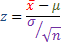1-proportion means test (Z-test)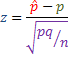2-sample means test (Z-test)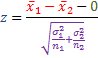2-sample means test (dependent samples)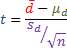Although the four equations look very different, they all have exactly the same structure: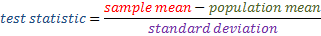The reason the formulas all look different is because we determine the population mean and standard deviation differently for different sample tests. But if you remember that all the test statistics have the same basic structure, you will find your calculations are a lot easier to figure out.

## What do probability distributions tell us?

A probability distribution can be almost completely described if we know its shape, its center and its variation. Most of Descriptive Statistics (typically the first semester) is about describing the shape, center and variation of our data set.

There are a lot of formulas to learn in your Stats class. Keep in mind that almost all of them in the first semester are used to help us characterize each type of distribution we learn.

Here are some examples:

Binomial Distribution—its shape, center and distribution are defined by:$p(x)= \displaystyle \binom{n}{x} p^x(1-p)^{n-x}; \; \mu = np; \; \sigma= \sqrt{np(1-p)}$

Geometric Distribution—its shape, center and distribution are defined by:$p(x)=p(1-p)^{x-1}; \; \mu = \dfrac{1}{p}; \; \sigma = \dfrac{\sqrt{1-p}}{p}$

Exponential Distribution—its shape, center and distribution are defined by:$p(x)= \dfrac{1}{\beta}e^{-x/\beta}; \; \mu = \beta; \; \sigma = \beta$

Where do these formulas come from? Well, we calculate the mean and standard deviation for a distribution just the way you do for a sample. But the algebra can get a little messy, and unless you are a statistics major, you probably don’t care. Just identify the type of distribution you have, and use the formulas to determine the mean and standard deviation.